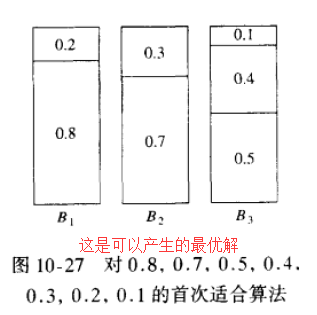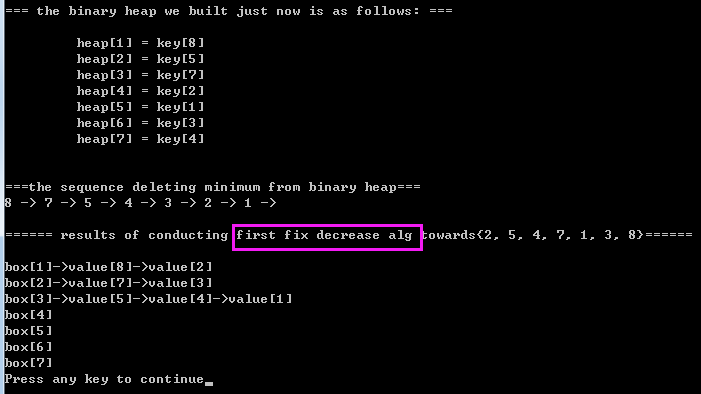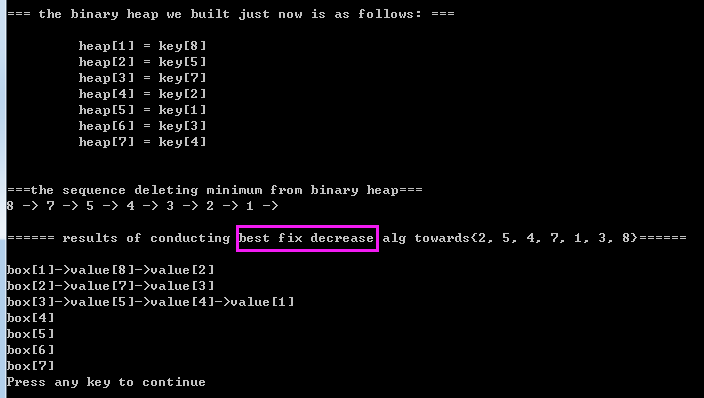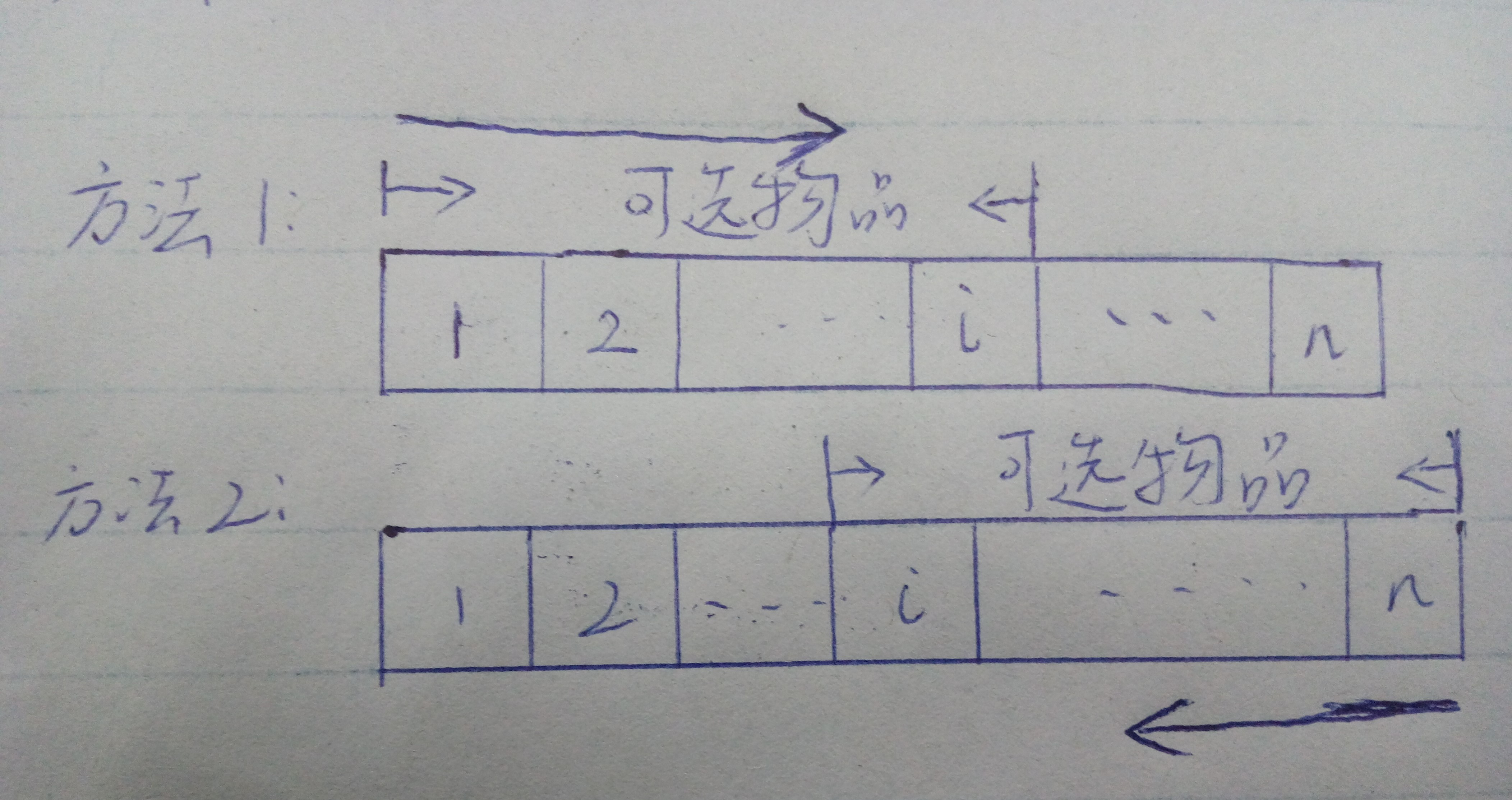• 【0】README0.1） 本文总结于 数据结构与算法分析， 源代码均为原创， 旨在 理解 “近似装箱问题（两种脱机算法实现）” 的idea 并用源代码加以实现； 0.2） 近似装箱问题的两种联机算法 分别是： 首次适合递减算法...
【0】README

0.1） 本文总结于 数据结构与算法分析， 源代码均为原创， 旨在 理解 “近似装箱问题（两种脱机算法实现）” 的idea 并用源代码加以实现；  0.2） 近似装箱问题的两种联机算法 分别是： 首次适合递减算法　和　最佳适合递减算法 ， 我们将依次给出源代码实现+算法描述； 0.3）联机算法+脱机算法

version1）联机装箱问题： 在这种问题中， 必须将每一件物品放入一个箱子后才处理下一件物品；（英语口语考试， 做完上一题，才能进入下一题作答）
version2）脱机装箱问题：在一个脱机装箱算法中， 我们做任何事情 都需要等到所有的输入数据全被读入后才进行；（一般的考试，你只需要在规定的时间做完题目即可，做题顺序不是我们所关心的） 0.3）不得不提的是：　我的源代码中，桶的容量设为了10，而不是１：原因是 由于 C语言的double类型的精度无法准确表示 小数值（它的有效位数是15~16位），所以，会使得最后的算法结果错误， 当然了对于容量为1的版本，我也写了源代码，参见：https://github.com/pacosonTang/dataStructure-algorithmAnalysis/tree/master/chapter10/p274_firstFitDecreaseOne， 有兴趣的朋友，可以down 下来，运行并测试一下；
【1】脱机算法相关

1.0）联机算法的主要问题：在于将大项物品装箱困难，　特别是当他们在输入的晚期出现的时候，　 1.1）围绕这个问题的自然方法：将各项物品排序，把最大的物品放在最先，此时我们可以应用首次适合算法或最佳适合算法，分别得到　“首次适合递减算法”　和　”最佳适合递减算法”； 1.2）看个荔枝（可以产生的最优解）：1.3）我们可以证明的结论有（Conclusion）：

C1）如果一种最优装箱法使用M 个箱子， 那么首次适合递减算法使用的箱子数目绝对不超过 （4M+1）/3；
C2）首先， 所有重量大于1/3 的项将被放入前M个箱子内， 这意味着， 在外加的箱子中所有各项的重量顶多是 1/3；
C3）在外加的箱子中 ， 物品的项数最多是 M-1；
C4）结合C2 和 C3， 我们发现， 外加的箱子最多可能需要 |(M-1)/3|（不小于（M-1）/3 的最小整数）个；
【2】source code + printing results（first fit decrease alg）

int main()
{
ElementTypePtr tempBox;
int tempKey;
int key = {2, 5, 4, 7, 1, 3, 8};
int i;

size = 7;
initBox(size);
surplus = buildBasicArray(size, 10); // building surplus array to store surplus value
tempBox = buildSingleElement();
bh = initBinaryHeap(size + 1);

for(i=0; i<size; i++)
{
tempBox->key = key[i];
insertHeap(*tempBox, bh);
}// building binary heap over

printBinaryHeap(bh);
printf("\n");
printf("\n===the sequence deleting minimum from binary heap===\n");
while(!isEmpty(bh))
{
tempKey = deleteMin(bh)->key;
printf("%d -> ", tempKey);
firstFixDecrease(tempKey);
}
printf("\n");
printBox(size);
return 0;
}

void firstFixDecrease(int key)
{
int i;
ElementTypePtr box;
ElementTypePtr temp;

box = buildSingleElement();
box->key = key; // build single box with key over

for(i=0; i<size; i++)
{
if(surplus[i] < key)
continue;
else
break;
}
temp = boxes[i] ;
while(temp->next)
temp = temp->next;
temp->next = box;
surplus[i] -= key;
}

2.3）printing results：【3】source code + printing results（best fit decrease alg）

int main()
{
ElementTypePtr tempBox;
int tempKey;
int key = {2, 5, 4, 7, 1, 3, 8};
int i;

size = 7;
initBox(size);
surplus = buildBasicArray(size, 10); // building surplus array to store surplus value
tempBox = buildSingleElement();
bh = initBinaryHeap(size + 1);

for(i=0; i<size; i++)
{
tempBox->key = key[i];
insertHeap(*tempBox, bh);
}// building binary heap over

printBinaryHeap(bh);
printf("\n");
printf("\n===the sequence deleting minimum from binary heap===\n");
while(!isEmpty(bh))
{
tempKey = deleteMin(bh)->key;
printf("%d -> ", tempKey);
bestFixDecrease(tempKey);
}
printf("\n");
printBox(size);
return 0;
}

void bestFixDecrease(int key)
{
int i;
ElementTypePtr box;
ElementTypePtr temp;
int minimum;
int miniIndex;

box = buildSingleElement();
box->key = key; // build single box with key over
miniIndex = 0;
minimum = 10;

for(i=0; i<size; i++)
{
if(surplus[i] < key)
continue;
if(surplus[i] - key < minimum)
{
minimum = surplus[i] - key;
miniIndex = i;
}
}

temp = boxes[miniIndex] ;
while(temp->next)
temp = temp->next;
temp->next = box;
surplus[miniIndex] -= key;
}

3.3）printing results：展开全文数据结构
• 问题描述：给定n种物品和一背包。物品i的重量为wi，其价值为vi， 背包容量为c。问应如何选择装入背包中的物品，使得背入背包的物品的总价值最大？解析：此问题形式化的描述是，给定c &gt; 0, wi, vi, 1 &lt;...
问题描述：给定n种物品和一背包。物品i的重量为wi，其价值为vi， 背包容量为c。问应如何选择装入背包中的物品，使得背入背包的物品的总价值最大？解析：此问题形式化的描述是，给定c > 0, wi, vi, 1 <= i <= n（c为背包容量）, 要找出一个n元0-1向量（x1, x2, ... , xn）,xi ∈ {0, 1}, 1 <= i <= n, 使得∑ wixi (i 从 1 到 n 求和）<= c ，而且∑ wixi (i 从 1 到 n 求和）达到最大。因此0-1背包问题是一个特殊的整数规划问题。方法1：递归关系：(这里与课本的描述不同个人感觉课本的“加”比较难理解, 这里用的“减”， 相信我继续看下去QAQ， 方法2用课本的方法“加”)设所给0-1背包问题的子问题的最优值为m[i][j], 即m[i][j]的含义是是在背包容量为j，可选物品为1, 2, 3, ..., i 时的0-1背包问题的最优值。由0-1背包问题的最优子结构性质，可建立计算m[i][j]的递归式如下：m[i][j] = max{m[i - 1][j], m[i - 1][j - w[i]] + v[i]}     j >= w[i];            = m[i - 1][j]                                                    j < w[i];递归关系流程化：1：如果不放入第 i 件物品，则问题转换为“前i - 1件物品放入容量为 j 的背包中”；2：如果放入第 i 件物品，则问题转化为“前i - 1件物品放入容量为 j - w[i] 的背包中”，此时的最大值为 max{m[i - 1][j], m[i - 1][j - w[i]] + v[i] }.代码：/*
输入样例 1:

3 10
4 3
5 4
6 5
输出为:

最优解为 : 11
选择的物品的序号为 :
2 3

输入样例 2:

5 10
6 2
3 2
5 6
4 5
6 4
输出为:

最优解为 : 15
选择的物品的序号为 :
1 2 5
*/
#include <bits/stdc++.h>
using namespace std;
const int MAX = 1024;
int n; //物品个数
int c; //包的容量
int value[MAX]; //物品的价值
int weight[MAX]; //物品的重量
int x[MAX]; //n元0-1向量
int m[MAX][MAX]; //解的容器
void Input()
{
scanf("%d %d", &n, &c);
for(int i = 1; i <= n; ++i)
scanf("%d %d", &value[i], &weight[i]);
}
//创建最优解
void Knapsack()
{
memset(m, 0, sizeof(m));
for(int i = 1; i <= n; ++i) //逐行填表，i表示当前可选物品数，j表示当前背包的容量, 也就是从低到顶。
{
for(int j = 1; j <= c; ++j)
{
if(j < weight[i])
m[i][j] = m[i - 1][j];
else
{
m[i][j] = max(m[i - 1][j], m[i - 1][j - weight[i]] + value[i]);
}
}
}
}
//获取最优解(即设法将求得的最优解输出出来)
void Traceback()
{
int cc = c;
for(int i = n; i > 1; --i)
{
if(m[i][cc] == m[i - 1][cc])
x[i] = 0;
else
{
x[i] = 1;
cc -= weight[i];
}
}
if(cc >= weight)
x = 1;

}
void Output()
{
cout << "最优解为 : " << m[n][c] << endl;
cout << "选择的物品的序号为 :" << endl;
for(int i = 1; i <= n; ++i)
if(x[i] == 1)
cout << i << " ";
cout << endl;
}
int main()
{
Input();
Knapsack();
Traceback();
Output();
}参考博客 ：https://www.cnblogs.com/vincently/p/4804225.html， 补充了Traceback()求解的过程， 完善了代码。方法2：（解释见代码注释）递归关系：设所给0-1背包问题的子问题的最优值为m[i][j], 即m[i][j]的含义是背包容量为j，可选物品为i, i + 1, ... n 时的0-1背包问题的最优值。由0-1背包问题的最优子结构性质，可建立计算m[i][j]的递归式如下：m[i][j] = max{m[i + 1][j], m[i + 1][j - w[i]] + v[i]}     j >= w[i];            = m[i + 1][j]                                                    j < w[i];递归关系流程化：1：如果不放入第 i 件物品，则问题转换为“i + 1, i + 2,  ..... , n件物品放入容量为 j 的背包中”；2：如果放入第 i 件物品，则问题转化为“i + 1, i + 2,  ..... , n 件物品放入容量为 j - w[i] 的背包中”，此时的最大值为 max{m[i + 1][j],  m[i + 1][j - w[i]] + v[i]}.与方法1的区别：请先务必先看懂方法1~。首先我们知道求最优解的过程就是填表m的过程。 方法1是从第一行开始填表m，而方法2是从第n行开始填表m即（两种方法都是是从底往上求解，不过方法一是可选物品从1-> i(从左到右)，方法二是可选物品从i -> n(从右向左）。这就是区别。而思路完全一样。请务必好好想想，然后就会恍然大悟٩(๑>◡<๑)۶代码：总的来说方法2比方法1运行速度快。/*
输入样例 1:

3 10
4 3
5 4
6 5
输出为:

最优解为 : 11
选择的物品的序号为 :
2 3

输入样例 2:

5 10
6 2
3 2
5 6
4 5
6 4
输出为:

最优解为 : 15
选择的物品的序号为 :
1 2 5
*/
#include <bits/stdc++.h>
using namespace std;
const int MAX = 1024;
int n; //物品个数
int c; //包的容量
int value[MAX]; //物品的价值
int weight[MAX]; //物品的重量
int x[MAX]; //n元0-1向量
int m[MAX][MAX]; //解的容器
void Input()
{
scanf("%d %d", &n, &c);
for(int i = 1; i <= n; ++i)
scanf("%d %d", &value[i], &weight[i]);
}
//创建最优解
void Knapsack()
{
int jMax = min(c, weight[n] - 1);

//这一块填最后m表的最后一行
/*
解释一下就是：“在可选的物品只有n即最后一个物品n，包的容量为c时” 的最优解。
第一个for循环：
容易知道在背包容量为0 ~ weight[n] - 1的时候背包是放不进去物品n的，如果背包
的容量小于物品n的质量，背包也是放不进去物品的，所以从weight[n] - 1 和 c 中
选择一个较小的，然后m[n][0:jMax]的值为零
第二个for循环：
自然可知当背包容量大于weigh[n]的时候,由于其可选则的物品只有 物品n，因此m[n][weight[n]:c]
的值全部为value[n].
*/
for(int j = 0; j <= jMax; ++j)
m[n][j] = 0;
for(int j = weight[n]; j <= c; ++j)
m[n][j] = value[n];

//这一块是填m表的2 ~ n - 1行，容易理解
for(int i = n - 1; i > 1; --i)
{
jMax = min(c, weight[i] - 1);
for(int j = 0; j <= jMax; ++j)
m[i][j] = m[i + 1][j];
for(int j = weight[i]; j <= c; ++j)
m[i][j] = max(m[i + 1][j], m[i + 1][j - weight[i]] + value[i]);
}

//这里是填m表的第一行，好好理解一下，不难，好好考虑一下 φ(>ω<*)
m[c] = m[c];
if(c >= weight)
m[c] = max(m[c], m[c - weight] + value);
}
//获取最优解(即设法将求得的最优解输出出来)
void Traceback()
{
int cc = c;
for(int i = 1; i < n; i++)
if(m[i][cc] == m[i + 1][cc])
x[i] = 0;
else
{
x[i] = 1;
cc -= weight[i];
}
x[n] = (m[n][cc]) ? 1 : 0;
}
void Output()
{
cout << "最优解为 : " << m[c] << endl;
cout << "选择的物品的序号为 :" << endl;
for(int i = 1; i <= n; ++i)
if(x[i] == 1)
cout << i << " ";
cout << endl;
}
int main()
{
Input();
Knapsack();
Traceback();
Output();
//    cout << "*******" << endl;
//    for(int i = 1; i <= n; ++i)
//    {
//        for(int j = 1; j <= c; ++j)
//            cout << m[i][j] << " ";
//        cout << endl;
//    }
}方法三：基于以方法一的路径压缩思路：定义m[j] : m[j]为背包容量为 j 时（注意不是剩余容量），背包装入的最大value。解题流程：遍历 i （可选物品为 1 ~ i），m[j] = max{ m[j], m[j - weight[i]] + value[i] }缺点：无法求出选中了哪些物品#include <bits/stdc++.h>
using namespace std;
const int MAX = 1024;
int n; //物品个数
int c; //包的容量
int value[MAX]; //物品的价值
int weight[MAX]; //物品的重量
int x[MAX]; //n元0-1向量
int m[MAX]; //解的容器
void Input()
{
scanf("%d %d", &n, &c);
for(int i = 1; i <= n; ++i)
scanf("%d %d", &value[i], &weight[i]);
}
//创建最优解
void Knapsack()
{
memset(m, 0, sizeof(m));
for(int i = 1; i <= n; ++i)
{
for(int j = c; j >= weight[i]; --j)
{
m[j] = max(m[j], m[j - weight[i]] + value[i]);
}
}
}
void Output()
{
cout << "最优解为 : " << m[c] << endl;
cout << endl;
}
int main()
{
Input();
Knapsack();

Output();
}

展开全文0-1背包问题 动态规划
• 首先回顾一下，协同过滤算法主要有两种，一种是基于用户的协同过滤算法（UserCF），另一种是基于物品的协同过滤算法（ItemCF）。 基于用户的协同过滤算法主要有两步： 1）找到和目标用户兴趣相似的用户集合  ...

首先回顾一下，协同过滤算法主要有两种，一种是基于用户的协同过滤算法（UserCF），另一种是基于物品的协同过滤算法（ItemCF）。

基于用户的协同过滤算法主要有两步：

1）找到和目标用户兴趣相似的用户集合

2）找到这个集合中的用户喜欢的，且目标用户没有听说过的物品推荐给目标用户。

基于物品的协同过滤算法主要有两步：

1）计算物品之间的相似度。

2）根据物品的相似度和用户的历史行为给用户生成推荐列表。

由此可以看出UserCF是推荐用户所在兴趣小组中的热点，更注重社会化，而ItemCF则是根据用户历史行为推荐相似物品，更注重个性化。所以UserCF一般用在新闻类网站中，如Digg,而ItemCF则用在其他非新闻类网站中，如Amazon,hulu等等。

因为在新闻类网站中，用户的兴趣爱好往往比较粗粒度，很少会有用户说只看某个话题的新闻，往往某个话题也不是天天会有新闻的。个性化新闻推荐更强盗新闻热点，热门程度和时效性是个性化新闻推荐的重点，个性化是补充，所以UserCF给用户推荐和他有相同兴趣爱好的人关注的新闻，这样在保证了热点和时效性的同时，兼顾了个性化。另外一个原因是从技术上考虑的，作为一种物品，新闻的更新非常快，而且实时会有新的新闻出现，而如果使用ItemCF的话，需要维护一张物品之间相似度的表，实际工业界这表一般是一天一更新的，这在新闻领域是万万不能接受的。

但是，在图书，电子商务和电影网站等方面，ItemCF则能更好的发挥作用。因为在这些网站中，用户的兴趣爱好一般是比较固定的，而且相比于新闻网站更细腻。在这些网站中，个性化推荐一般是给用户推荐他自己领域的相关物品。另外，这些网站的物品数量更新速度不快，一天一次更新可以接受。而且在这些网站中，用户数量往往远远大于物品数量，从存储的角度来讲，UserCF需要消耗更大的空间复杂度，另外，ItemCF可以方便的提供推荐理由，增加用户对推荐系统的信任度，所以更适合这些网站。

转自：http://blog.csdn.net/wangyuquanliuli/article/details/37603487


展开全文推荐系统 协同过滤 用户协同过滤
• 0-1 背包问题：给定 n 种物品和一个容量为 C 的背包，物品 i 的重量是 wi，其价值为 vi 。 问：应该如何选择装入背包的物品，使得装入背包中的物品的总价值最大？ 分析一波，面对每个物品，我们只有选择拿取...


0-1 背包问题：给定 n 种物品和一个容量为 C 的背包，物品 i 的重量是 wi，其价值为 vi 。

问：应该如何选择装入背包的物品，使得装入背包中的物品的总价值最大？

分析一波，面对每个物品，我们只有选择拿取或者不拿两种选择，不能选择装入某物品的一部分，也不能装入同一物品多次。

解决办法：声明一个 大小为  m[n][c] 的二维数组，m[ i ][ j ] 表示 在面对第 i 件物品，且背包容量为  j 时所能获得的最大价值 ，那么我们可以很容易分析得出 m[i][j] 的计算方法，

（1）. j < w[i] 的情况，这时候背包容量不足以放下第 i 件物品，只能选择不拿

m[ i ][ j ] = m[ i-1 ][ j ]

（2）. j>=w[i] 的情况，这时背包容量可以放下第 i 件物品，我们就要考虑拿这件物品是否能获取更大的价值。

如果拿取，m[ i ][ j ]=m[ i-1 ][ j-w[ i ] ] + v[ i ]。 这里的m[ i-1 ][ j-w[ i ] ]指的就是考虑了i-1件物品，背包容量为j-w[i]时的最大价值，也是相当于为第i件物品腾出了w[i]的空间。

如果不拿，m[ i ][ j ] = m[ i-1 ][ j ] , 同（1）

究竟是拿还是不拿，自然是比较这两种情况那种价值最大。

由此可以得到状态转移方程：

if(j>=w[i])
m[i][j]=max(m[i-1][j],m[i-1][j-w[i]]+v[i]);
else
m[i][j]=m[i-1][j]; 

例：0-1背包问题。在使用动态规划算法求解0-1背包问题时，使用二维数组m[i][j]存储背包剩余容量为j，可选物品为i、i+1、……、n时0-1背包问题的最优值。绘制

价值数组v = {8, 10, 6, 3, 7, 2}，

重量数组w = {4, 6, 2, 2, 5, 1}，

背包容量C = 12时对应的m[i][j]数组。

0

1

2

3

4

5

6

7

8

9

10

11

12

1

0

0

0

8

8

8

8

8

8

8

8

8

2

0

0

0

8

8

10

10

10

10

18

18

18

3

0

6

6

8

8

14

14

16

16

18

18

24

4

0

6

6

9

9

14

14

17

17

19

19

24

5

0

6

6

9

9

14

14

17

17

19

21

24

6

2

6

8

9

11

14

16

17

19

19

21

24
(第一行和第一列为序号，其数值为0)如m,在面对第二件物品，背包容量为6时我们可以选择不拿，那么获得价值仅为第一件物品的价值8，如果拿，就要把第一件物品拿出来，放第二件物品，价值10，那我们当然是选择拿。m=m+10=0+10=10;依次类推，得到m就是考虑所有物品，背包容量为C时的最大价值。#include <iostream>
#include <cstring>
using namespace std;

const int N=15;

int main()
{
int v[N]={0,8,10,6,3,7,2};
int w[N]={0,4,6,2,2,5,1};

int m[N][N];
int n=6,c=12;
memset(m,0,sizeof(m));
for(int i=1;i<=n;i++)
{
for(int j=1;j<=c;j++)
{
if(j>=w[i])
m[i][j]=max(m[i-1][j],m[i-1][j-w[i]]+v[i]);

else
m[i][j]=m[i-1][j];
}
}

for(int i=1;i<=n;i++)
{
for(int j=1;j<=c;j++)
{
cout<<m[i][j]<<' ';
}
cout<<endl;
}

return 0;
}  

到这一步，可以确定的是可能获得的最大价值，但是我们并不清楚具体选择哪几样物品能获得最大价值。

另起一个 x[ ] 数组，x[i]=0表示不拿，x[i]=1表示拿。

m[n][c]为最优值，如果m[n][c]=m[n-1][c] ,说明有没有第n件物品都一样，则x[n]=0 ; 否则 x[n]=1。当x[n]=0时，由x[n-1][c]继续构造最优解；当x[n]=1时，则由x[n-1][c-w[i]]继续构造最优解。以此类推，可构造出所有的最优解。（这段全抄算法书，实在不知道咋解释啊。。）
void traceback()
{
for(int i=n;i>1;i--)
{
if(m[i][c]==m[i-1][c])
x[i]=0;
else
{
x[i]=1;
c-=w[i];
}
}
x=(m[c]>0)?1:0;
}  例：

某工厂预计明年有A、B、C、D四个新建项目，每个项目的投资额Wk及其投资后的收益Vk如下表所示，投资总额为30万元，如何选择项目才能使总收益最大？

Project

Wk

Vk

A

15

12

B

10

8

C

12

9

D

8

5

结合前面两段代码#include <iostream>
#include <cstring>
using namespace std;

const int N=150;

int v[N]={0,12,8,9,5};
int w[N]={0,15,10,12,8};
int x[N];
int m[N][N];
int c=30;
int n=4;
void traceback()
{
for(int i=n;i>1;i--)
{
if(m[i][c]==m[i-1][c])
x[i]=0;
else
{
x[i]=1;
c-=w[i];
}
}
x=(m[c]>0)?1:0;
}

int main()
{
memset(m,0,sizeof(m));
for(int i=1;i<=n;i++)
{
for(int j=1;j<=c;j++)
{
if(j>=w[i])
m[i][j]=max(m[i-1][j],m[i-1][j-w[i]]+v[i]);

else
m[i][j]=m[i-1][j];
}
}
traceback();
for(int i=1;i<=n;i++)
cout<<x[i];
return 0;
}  

输出x[i]数组：0111，输出m：22。

得出结论：选择BCD三个项目总收益最大，为22万元。

不过这种算法只能得到一种最优解，并不能得出所有的最优解。
转载自：https://blog.csdn.net/xp731574722/article/details/70766804

展开全文• 在浅谈语音助手的对话管理与策略制定中提到过导购场景的推荐型bot，这类bot关注的问题在于如何与用户交互以明确用户的意图，以及如何对匹配物品排序和优化交互过程。本文针对这些问题，谈谈关于对话交互与推荐系统的...算法 机器学习 自然语言处理 深度学习
• 本文首先介绍了推荐系统的发展历史，及目前常用的几推荐算法的介绍与比较，然后以基于物品的协同过滤算法为基础，详细介绍图书推荐系统的构建。在该系统中，主要功能分为用户功能和图书推荐功能...协同过滤算法
• 可选物品两种属性，分别是重量和重要性。二维列表如下（第一位是物品名称，第二位是重要性，第三位是重量）： [["knife","10","1"],["Beans","20","5"],["Potatoes","15","10"],["Onions","2","1"],["Sleeping ...python
• 一直以来，推荐系统使用的都是使用Collaborative Filtering（协同过滤）算法：利用用户和物品间的相似关系，计算用户对物品的喜好程度。然后将预计用户最喜欢的前N个物品推荐给它，以实现个性化推荐。可以看到，这...推荐系统 排序
• 校园闲置物品交易平台的设计与实现（二手交易平台） 包含论文，APP及网站系统三个内容。 首先是论文的部分： 首先是APP的展示 代码： public class CommentInfo implements Parcelable { private int cId...php android html mysql
• 在协同过滤中，有两种主流方法：基于用户的协同过滤，和基于物品的协同过滤。具体怎么来阐述他们的原理呢，看个图大家就明白了 基于用户的 CF 的基本思想相当简单，基于用户对物品的偏好找到相邻邻居用户，...推荐系统 协同过滤 基于用户的协同过滤
• ## 结合个人经历总结的前端入门方法

万次阅读 多人点赞 2015-12-04 16:08:41
结合个人经历总结的前端入门方法，总结从零基础到具备前端基本技能的道路、学习方法、资料。由于能力有限，不能保证面面俱到，只是作为入门参考，面向初学者，让初学者少走弯路。 互联网的快速发展和激烈竞争，用户...前端 前端开发 web前端 web开发
• 挖局用户曾经喜欢的物品，推荐类似的产品 利用已知的用户自己的偏好，兴趣等属性和物品内容属性做匹配 将用户的个人信息的特征和内容对象的特征匹配，结果就是用户对某个对象感兴趣的程度 基于内容推荐的层次结构* ...
• 1. 前言: 为什么会有该系列? 最近，打算写《零基础入门推荐系统》系列，为了系统地介绍推荐系统知识，以及加强基础的实践能力。 该系列将结合一些书籍，比如项亮的《推荐系统...对用户uuu推荐NNN个物品（记为R(u)R(u
• MessyTable是商汤与新加坡南洋理工大学联合制作的大规模多相机通用物品场景数据集。针对现实生活中多相机系统应用的难点，如相似相同的物品、密集遮挡、大角度差等问题，我们设计了大量真实、有趣又极富挑战的场景：...算法 python 机器学习 人工智能 深度学习
• 那些还不能理解虚拟物品也可作为商品交易的人们，那些还没有发觉中国虚拟物品交易市场已经渐渐成形的人们。其心中的误区仅在于，他们总以为游戏仅仅是娱乐，而实际上游戏已是社区、游戏已是生活，游戏已是无边无际的...exchange 服务器
• 上篇博客我们说Spring web Flow与业务结合的方式主要有三，下面我们主要介绍一下第三的应用方式 3，执行到 元素SpringWeb Flow 中的这个 是专为执行业务逻辑而设的 state 。如果某个应用的业务逻辑代码既不适合...
• 结合美团下单率预测详解机器学习中的数据清洗与特征处理 发表于22小时前| 1632次阅读| 来源美团| 4 条评论| 作者caohao 机器学习数据挖掘可视化特征处理特征降维美团 摘要：本文主要介绍在美团的...机器学习
• 很明显的是，对于第i件物品，只有取或者不取两种状态，那么f[i][j]就是： 下面就很容易给出代码 ;font-size:14px;">for(int i = 1 ; i ; i ++) { for(int j = 0 ; j ; j ++) f[i][j] = max...
•  至此多提一句，最初对于3D物品的深度神经网络检测是由2015年提出的篇论文解决的。 1、将点云数据投影到二维平面。此方式不直接处理三维的点云数据，而是先将点云投影到某些特定视角再处理，如前视视角和...目标识别 语义分割
• 3、iOS11及其以上系统一般来说AR应用都有个步骤： 1、处理、追踪、了解现实世界的环境 2、渲染、展示场景的虚拟物件第一步是透过追踪引擎处理，也就是ARKit。 第二步是通过渲染引擎处理，比如SceneKit被用来...coreml ios
• 本文主要是参考Martion Fowler所著的《企业应用架构模式》与Eric Evans所著的《领域驱动设计》这本泰山之作，加上本人在近年实际的工作过程中开发SOA系统所认识到的问题所写的一篇文章，欢迎各位点评。 最后节...soa 领域模型 service class linq
• 目录 1. 概述 1.1 协同过滤 1.2 相似度的计算 ...2.4 案列3：基于物品推荐 3. 推荐系统的冷启动问题 ① 用户冷启动 ② 系统冷启动　 ③ 物品冷启动 1. 概述 买了一个手机，再次刷新会出现类似的产...
• 最近*海*原有系统需要进行改进，可能会将Flex改为Flex与jsp相结合的方式，好发挥两者的优势。这天在做html页面，页面内容主要展现在标签页中，其基础效果图如下所示：  为增强用户对标签页的体验效果，增加...html function div iframe javascript
• ## [C#]23种设计模式

千次阅读 2013-12-06 09:11:14
在工厂方法模式中，工厂方法用来创建客户所需要的产品，同时还向客户隐藏了哪具体产品类将被实例化这一细节。工厂方法模式的核心是一个抽象工厂类，各种具体工厂类通过抽象工厂类将工厂方法继承下来。如此使得客户...设计模式
• 目前推荐系统研宄的主要趋势是从单一的、独立的推荐系统算法逐渐向组合多种推荐算法形成混合式的综合推荐算法方向发展，越来越多的结合用户标签数据、社交网络数据、上下文信息、地理位置信息。群体推荐也成为一个...推荐算法
• 1、引言 Join是SQL语句中的常用操作，...SparkSQL作为大数据领域的SQL实现，自然也对Join操作做了不少优化，今天主要看一下在SparkSQL中对于Join，常见的3实现。 2 、Spark SQL中Join常用的实现 2.1 、Broa...hive
• 本章会从用户的评价类型开始讨论，包括显式...基于用户的协同过滤基于物品的协同过滤修正的余弦相似度Slope One算法Slope One的Python实现MovieLens数据 第二章中我们学习了协同过滤和推荐系统的基本知识，其中讲述
• 论文提到了两种，一种是 Weighted Hierarchical Partitionr，它的思想和加权的 K-means 算法一样，而权重是通过给训练样例到中心的距离一个根据 label 预测准确度得出的 weight，即如果在样本上打分函数表现的好(与...推荐系统 CTR预估
• 推荐系统现在的应用已经非常广泛了，协同过滤（CF）是一常见的推荐系统，仅根据用户过去的行为来进行推荐，不需要domain knowledge，也不需要进行大规模的数据收集。CF又分为 Neighborhood model （比如User-...推荐系统 人工智能 大数据 机器学习 数据挖掘...